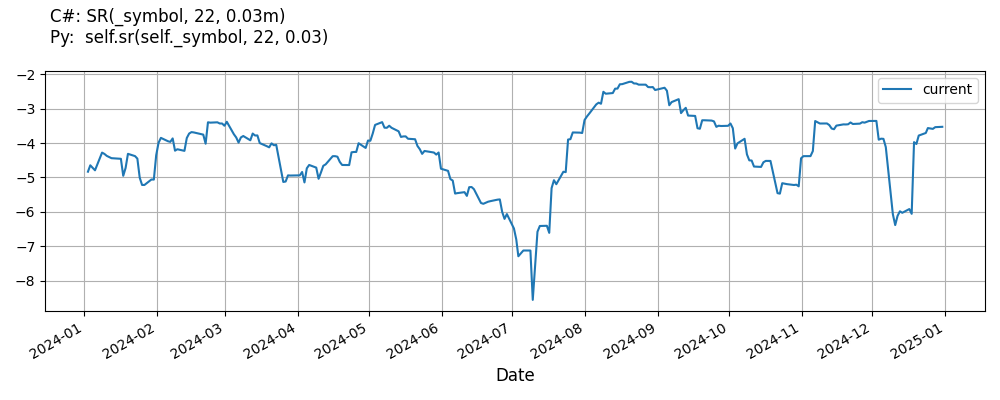# Supported Indicators

## Sharpe Ratio

### Introduction

Calculation of the Sharpe Ratio (SR) developed by William F. Sharpe. Reference: https://www.investopedia.com/articles/07/sharpe_ratio.asp Formula: S(x) = (Rx - Rf) / stdDev(Rx) Where: S(x) - sharpe ratio of x Rx - average rate of return for x Rf - risk-free rate

To view the implementation of this indicator, see the LEAN GitHub repository.

### Using SR Indicator

To create an automatic indicators for SharpeRatio, call the SR helper method from the QCAlgorithm class. The SR method creates a SharpeRatio object, hooks it up for automatic updates, and returns it so you can used it in your algorithm. In most cases, you should call the helper method in the Initialize method.

public class SharpeRatioAlgorithm : QCAlgorithm
{
private Symbol _symbol;
private SharpeRatio _sr;

public override void Initialize()
{
_sr = SR(_symbol, 22, 0.03);
}

public override void OnData(Slice data)
{
{
// The current value of _sr is represented by itself (_sr)
// or _sr.Current.Value
Plot("SharpeRatio", "sr", _sr);

}
}
}
class SharpeRatioAlgorithm(QCAlgorithm):
def Initialize(self) -> None:
self.sr = self.SR(self.symbol, 22, 0.03)

def OnData(self, slice: Slice) -> None:
# The current value of self.sr is represented by self.sr.Current.Value
self.Plot("SharpeRatio", "sr", self.sr.Current.Value)



The following reference table describes the SR method:

### SR()1/1

            SharpeRatio QuantConnect.Algorithm.QCAlgorithm.SR (
Symbol                           symbol,
Int32                            sharpePeriod,
*Nullable<Decimal>         riskFreeRate,
*Nullable<Resolution>      resolution,
*Func<IBaseData, Decimal>  selector
)


Creates a new SharpeRatio indicator.

If you don't provide a resolution, it defaults to the security resolution. If you provide a resolution, it must be greater than or equal to the resolution of the security. For instance, if you subscribe to hourly data for a security, you should update its indicator with data that spans 1 hour or longer.

You can manually create a SharpeRatio indicator, so it doesn't automatically update. Manual indicators let you update their values with any data you choose.

Updating your indicator manually enables you to control when the indicator is updated and what data you use to update it. To manually update the indicator, call the Update method with time/number pair, or an IndicatorDataPoint. The indicator will only be ready after you prime it with enough data.

public class SharpeRatioAlgorithm : QCAlgorithm
{
private Symbol _symbol;
private SharpeRatio _sr;

public override void Initialize()
{
_sr = new SharpeRatio(22, 0.03);
}

public override void OnData(Slice data)
{
if (data.Bars.TryGeValue(_symbol, out var bar))
{
_sr.Update(bar.EndTime, bar.Close);
}

{
// The current value of _sr is represented by itself (_sr)
// or _sr.Current.Value
Plot("SharpeRatio", "sr", _sr);

}
}
}
class SharpeRatioAlgorithm(QCAlgorithm):
def Initialize(self) -> None:
self.sr = SharpeRatio(22, 0.03)

def OnData(self, slice: Slice) -> None:
bar = slice.Bars.get(self.symbol)
if bar:
self.sr.Update(bar.EndTime, bar.Close)

# The current value of self.sr is represented by self.sr.Current.Value
self.Plot("SharpeRatio", "sr", self.sr.Current.Value)



To register a manual indicator for automatic updates with the security data, call the RegisterIndicator method.

public class SharpeRatioAlgorithm : QCAlgorithm
{
private Symbol _symbol;
private SharpeRatio _sr;

public override void Initialize()
{
_sr = new SharpeRatio(22, 0.03);
RegisterIndicator(_symbol, _sr, Resolution.Daily);
}

public override void OnData(Slice data)
{
{
// The current value of _sr is represented by itself (_sr)
// or _sr.Current.Value
Plot("SharpeRatio", "sr", _sr);

}
}
}
class SharpeRatioAlgorithm(QCAlgorithm):
def Initialize(self) -> None:
self.sr = SharpeRatio(22, 0.03)
self.RegisterIndicator(self.symbol, self.sr, Resolution.Daily)

def OnData(self, slice: Slice) -> None:
# The current value of self.sr is represented by self.sr.Current.Value
self.Plot("SharpeRatio", "sr", self.sr.Current.Value)



The following reference table describes the SharpeRatio constructor:

### SharpeRatio()1/6

            SharpeRatio QuantConnect.Indicators.SharpeRatio (
string                      name,
int                         period,
IRiskFreeInterestRateModel  riskFreeRateModel
)


Creates a new Sharpe Ratio indicator using the specified periods.

### SharpeRatio()2/6

            SharpeRatio QuantConnect.Indicators.SharpeRatio (
int                         period,
IRiskFreeInterestRateModel  riskFreeRateModel
)


Creates a new Sharpe Ratio indicator using the specified periods.

### SharpeRatio()3/6

            SharpeRatio QuantConnect.Indicators.SharpeRatio (
int       period,
PyObject  riskFreeRateModel
)


Creates a new Sharpe Ratio indicator using the specified period using a Python risk free rate model.

### SharpeRatio()4/6

            SharpeRatio QuantConnect.Indicators.SharpeRatio (
int       period,
PyObject  riskFreeRateModel
)


Creates a new Sharpe Ratio indicator using the specified period using a Python risk free rate model.

### SharpeRatio()5/6

            SharpeRatio QuantConnect.Indicators.SharpeRatio (
string    name,
int       period,
*decimal  riskFreeRate
)


Creates a new Sharpe Ratio indicator using the specified periods.

### SharpeRatio()6/6

            SharpeRatio QuantConnect.Indicators.SharpeRatio (
int       period,
*decimal  riskFreeRate
)


Creates a new SharpeRatio indicator using the specified periods.

### Visualization

The following image shows plot values of selected properties of SharpeRatio using the plotly library.You can also see our Videos. You can also get in touch with us via Discord.